login  home  contents  what's new  discussion  bug reports help  links  subscribe  changes  refresh  edit

# Edit detail for SandBoxInequation revision 8 of 11

 1 2 3 4 5 6 7 8 9 10 11 Editor: Bill Page Time: 2008/06/11 07:55:45 GMT-7 Note: Relations package

```changed:
-)abbrev domain NE Inequation
)abbrev domain NEQ Inequation

changed:
-)abbrev domain LT Inequality
)abbrev domain INEQ Inequality
```

```spad)abbrev domain NEQ Inequation
++ Author: Bill Page
++ Based on: Equation by Stephen M. Watt, enhancements by Johannes Grabmeier
++ Date Created: June 2008
++ Basic Operations: ~=
++ Related Domains: Equation
++ Also See:
++ AMS Classifications:
++ Keywords: inequation
++ Examples:
++ References:
++ Description:
++   Inequations as mathematical objects.  All properties of the basis domain,
++   e.g. being an abelian group are carried over the equation domain, by
++   performing the structural operations on the left and on the
++   right hand side.
--   The interpreter translates "~=" to "inequation".  Otherwise, it will
--   find a modemap for "~=" in the domain of the arguments.
Inequation(S: Type): public == private where
public ==> Type with
"~=": (S, S) -> \$
++ a~=b creates an inequation.
inequation: (S, S) -> \$
++ inequation(a,b) creates an inequation.
swap: \$ -> \$
++ swap(neq) interchanges left and right hand side of inequation neq.
lhs: \$ -> S
++ lhs(neq) returns the left hand side of inequation neq.
rhs: \$ -> S
++ rhs(neq) returns the right hand side of inequation neq.
map: (S -> S, \$) -> \$
++ map(f,neq) constructs a new inequation by applying f to both
++ sides of neq. (f must be an injection)
if S has InnerEvalable(Symbol,S) then
InnerEvalable(Symbol,S)
if S has SetCategory then
SetCategory
CoercibleTo Boolean
if S has Evalable(S) then
eval: (\$, Equation S) -> \$
++ eval(neq, x=f) replaces x by f in inequation neq.
eval: (\$, List Equation S) -> \$
++ eval(neq, [x1=v1, ... xn=vn]) replaces xi by vi in inequation neq.
if S has AbelianSemiGroup then
"+": (S, \$) -> \$
++ x+neq produces a new inequation by adding x to both sides of
++ inequation neq.
"+": (\$, S) -> \$
++ neq+x produces a new inequation by adding x to both sides of
++ inequation neq.
if S has AbelianGroup then
"-": \$ -> \$
leftZero : \$ -> \$
++ leftZero(neq) subtracts the left hand side.
rightZero : \$ -> \$
++ rightZero(neq) subtracts the right hand side.
"-": (S, \$) -> \$
++ x-neq produces a new equation by subtracting both sides of
++ equation neq from x.
"-": (\$, S) -> \$
++ neq-x produces a new equation by subtracting x from  both sides of
++ equation neq.
if S has Monoid then
recip: \$ -> Union(\$,"failed")
leftOne : \$ -> Union(\$,"failed")
++ leftOne(neq) divides by the left hand side, if possible.
rightOne : \$ -> Union(\$,"failed")
++ rightOne(neq) divides by the right hand side, if possible.
if S has Group then
leftOne : \$ -> Union(\$,"failed")
++ leftOne(neq) divides by the left hand side.
rightOne : \$ -> Union(\$,"failed")
++ rightOne(neq) divides by the right hand side.
if S has IntegralDomain then
factorAndSplit : \$ -> List \$
++ factorAndSplit(neq) make the right hand side 0 and
++ factors the new left hand side. Each factor is equated
++ to 0 and put into the resulting list without repetitions.
if S has ExpressionSpace then
subst: (\$, Equation S) -> \$
++ subst(neq1,eq2) substitutes eq2 into both sides of neq1
++ the lhs of eq2 should be a kernel
Rep := Record(lhs: S, rhs: S)
neq1,neq2, neq: \$
eq2: Equation S
s : S
if S has IntegralDomain then
factorAndSplit neq ==
(S has factor : S -> Factored S) =>
neq0 := rightZero neq
[inequation(rcf.factor,0) for rcf in factors factor lhs neq0]
[neq]
l:S ~= r:S      == [l, r]
inequation(l, r) == [l, r]    -- hack!  See comment above.
lhs neq  == neq.lhs
rhs neq  == neq.rhs
swap neq == [rhs neq, lhs neq]
map(fn, neq)   == inequation(fn(neq.lhs), fn(neq.rhs))
if S has InnerEvalable(Symbol,S) then
s:Symbol
ls:List Symbol
x:S
lx:List S
eval(neq,s,x) == eval(neq.lhs,s,x) ~= eval(neq.rhs,s,x)
eval(neq,ls,lx) == eval(neq.lhs,ls,lx) ~= eval(neq.rhs,ls,lx)
if S has Evalable(S) then
eval(neq1:\$, eqn2:Equation S):\$ ==
eval(neq1.lhs, eqn2) ~= eval(neq1.rhs, eqn2)
eval(neq1:\$, leqn2:List Equation S):\$ ==
eval(neq1.lhs, leqn2) ~= eval(neq1.rhs, leqn2)
if S has SetCategory then
neq1 = neq2 == (neq1.lhs = neq2.lhs)@Boolean and
(neq1.rhs = neq2.rhs)@Boolean
coerce(neq:\$):OutputForm == blankSeparate([neq.lhs::OutputForm, "~=", neq.rhs::OutputForm])\$OutputForm
coerce(neq:\$):Boolean == neq.lhs ~= neq.rhs
if S has AbelianSemiGroup then
s + neq2 == s+neq2.lhs ~= s+neq2.rhs
neq1 + s == neq1.lhs+s ~= neq1.rhs+s
if S has AbelianGroup then
- neq == -neq.lhs ~= -neq.rhs
s - neq2 == s-neq2.lhs ~= s-neq2.rhs
neq1 - s == neq1.lhs-s ~= neq1.rhs-s
leftZero neq == 0 ~= rhs neq - lhs neq
rightZero neq == lhs neq - rhs neq ~= 0
if S has Monoid then
recip neq ==
(lh := recip lhs neq) case "failed" => "failed"
(rh := recip rhs neq) case "failed" => "failed"
[lh :: S, rh :: S]
leftOne neq ==
(re := recip lhs neq) case "failed" => "failed"
1 ~= rhs neq * re
rightOne neq ==
(re := recip rhs neq) case "failed" => "failed"
lhs neq * re ~= 1
if S has Group then
leftOne neq == 1 ~= rhs neq * inv rhs neq
rightOne neq == lhs neq * inv rhs neq ~= 1
if S has IntegralDomain then
factorAndSplit neq ==
(S has factor : S -> Factored S) =>
neq0 := rightZero neq
[inequation(rcf.factor,0) for rcf in factors factor lhs neq0]
(S has Polynomial Integer) =>
neq0 := rightZero neq
MF ==> MultivariateFactorize(Symbol, IndexedExponents Symbol, _
Integer, Polynomial Integer)
p : Polynomial Integer := (lhs neq0) pretend Polynomial Integer
[inequation((rcf.factor) pretend S,0) for rcf in factors factor(p)\$MF]
[neq]
if S has ExpressionSpace then
subst(neq1,eq2) ==
[subst(lhs neq1,eq2),subst(rhs neq1,eq2)]```
```   Compiling FriCAS source code from file
old system compiler.
NEQ abbreviates domain Inequation
processing macro definition public ==> -- the constructor category
processing macro definition private ==> -- the constructor capsule
------------------------------------------------------------------------
initializing NRLIB NEQ for Inequation
compiling into NRLIB NEQ
****** Domain: S already in scope
augmenting S: (IntegralDomain)
augmenting \$: (SIGNATURE \$ factorAndSplit ((List \$) \$))
compiling exported factorAndSplit : \$ -> List \$
augmenting S: (SIGNATURE S factor ((Factored S) S))
Time: 0.04 SEC.
compiling exported ~= : (S,S) -> \$
NEQ;~=;2S\$;2 is replaced by CONS
Time: 0 SEC.
compiling exported inequation : (S,S) -> \$
NEQ;inequation;2S\$;3 is replaced by CONS
Time: 0 SEC.
compiling exported lhs : \$ -> S
NEQ;lhs;\$S;4 is replaced by QCAR
Time: 0 SEC.
compiling exported rhs : \$ -> S
NEQ;rhs;\$S;5 is replaced by QCDR
Time: 0 SEC.
compiling exported swap : \$ -> \$
Time: 0 SEC.
compiling exported map : (S -> S,\$) -> \$
Time: 0 SEC.
****** Domain: S already in scope
augmenting S: (InnerEvalable (Symbol) S)
compiling exported eval : (\$,Symbol,S) -> \$
Time: 0 SEC.
compiling exported eval : (\$,List Symbol,List S) -> \$
Time: 0.01 SEC.
****** Domain: S already in scope
augmenting S: (Evalable S)
compiling exported eval : (\$,Equation S) -> \$
Time: 0.06 SEC.
compiling exported eval : (\$,List Equation S) -> \$
Time: 0.01 SEC.
****** Domain: S already in scope
augmenting S: (SetCategory)
compiling exported = : (\$,\$) -> Boolean
Time: 0 SEC.
compiling exported coerce : \$ -> OutputForm
Time: 0 SEC.
compiling exported coerce : \$ -> Boolean
Time: 0.01 SEC.
****** Domain: S already in scope
augmenting S: (AbelianSemiGroup)
augmenting \$: (SIGNATURE \$ + (\$ S \$))
augmenting \$: (SIGNATURE \$ + (\$ \$ S))
compiling exported + : (S,\$) -> \$
Time: 0 SEC.
compiling exported + : (\$,S) -> \$
Time: 0 SEC.
****** Domain: S already in scope
augmenting S: (AbelianGroup)
augmenting \$: (SIGNATURE \$ - (\$ \$))
augmenting \$: (SIGNATURE \$ leftZero (\$ \$))
augmenting \$: (SIGNATURE \$ rightZero (\$ \$))
augmenting \$: (SIGNATURE \$ - (\$ S \$))
augmenting \$: (SIGNATURE \$ - (\$ \$ S))
compiling exported - : \$ -> \$
Time: 0 SEC.
compiling exported - : (S,\$) -> \$
Time: 0 SEC.
compiling exported - : (\$,S) -> \$
Time: 0 SEC.
compiling exported leftZero : \$ -> \$
Time: 0 SEC.
compiling exported rightZero : \$ -> \$
Time: 0 SEC.
****** Domain: S already in scope
augmenting S: (Monoid)
augmenting \$: (SIGNATURE \$ recip ((Union \$ failed) \$))
augmenting \$: (SIGNATURE \$ leftOne ((Union \$ failed) \$))
augmenting \$: (SIGNATURE \$ rightOne ((Union \$ failed) \$))
compiling exported recip : \$ -> Union(\$,failed)
Time: 0.01 SEC.
compiling exported leftOne : \$ -> Union(\$,failed)
Time: 0 SEC.
compiling exported rightOne : \$ -> Union(\$,failed)
Time: 0 SEC.
****** Domain: S already in scope
augmenting S: (Group)
augmenting \$: (SIGNATURE \$ leftOne ((Union \$ failed) \$))
augmenting \$: (SIGNATURE \$ rightOne ((Union \$ failed) \$))
compiling exported leftOne : \$ -> Union(\$,failed)
Time: 0 SEC.
compiling exported rightOne : \$ -> Union(\$,failed)
Time: 0 SEC.
****** Domain: S already in scope
augmenting S: (IntegralDomain)
augmenting \$: (SIGNATURE \$ factorAndSplit ((List \$) \$))
compiling exported factorAndSplit : \$ -> List \$
augmenting S: (SIGNATURE S factor ((Factored S) S))
extension of ##1 to (Polynomial (Integer)) ignored
processing macro definition MF ==> MultivariateFactorize(Symbol,IndexedExponents Symbol,Integer,Polynomial Integer)
Time: 0.22 SEC.
****** Domain: S already in scope
augmenting S: (ExpressionSpace)
augmenting \$: (SIGNATURE \$ subst (\$ \$ (Equation S)))
compiling exported subst : (\$,Equation S) -> \$
Time: 0 SEC.
****** Domain: S already in scope
augmenting S: (Evalable S)
****** Domain: S already in scope
augmenting S: (SetCategory)
augmenting \$: (SIGNATURE \$ eval (\$ \$ (Equation S)))
augmenting \$: (SIGNATURE \$ eval (\$ \$ (List (Equation S))))
****** Domain: S already in scope
augmenting S: (AbelianGroup)
augmenting \$: (SIGNATURE \$ - (\$ \$))
augmenting \$: (SIGNATURE \$ leftZero (\$ \$))
augmenting \$: (SIGNATURE \$ rightZero (\$ \$))
augmenting \$: (SIGNATURE \$ - (\$ S \$))
augmenting \$: (SIGNATURE \$ - (\$ \$ S))
****** Domain: S already in scope
augmenting S: (AbelianSemiGroup)
augmenting \$: (SIGNATURE \$ + (\$ S \$))
augmenting \$: (SIGNATURE \$ + (\$ \$ S))
****** Domain: S already in scope
augmenting S: (ExpressionSpace)
augmenting \$: (SIGNATURE \$ subst (\$ \$ (Equation S)))
****** Domain: S already in scope
augmenting S: (InnerEvalable (Symbol) S)
****** Domain: S already in scope
augmenting S: (IntegralDomain)
augmenting \$: (SIGNATURE \$ factorAndSplit ((List \$) \$))
****** Domain: S already in scope
augmenting S: (Monoid)
augmenting \$: (SIGNATURE \$ recip ((Union \$ failed) \$))
augmenting \$: (SIGNATURE \$ leftOne ((Union \$ failed) \$))
augmenting \$: (SIGNATURE \$ rightOne ((Union \$ failed) \$))
****** Domain: S already in scope
augmenting S: (SetCategory)
(time taken in buildFunctor:  0)
;;;     ***       |Inequation| REDEFINED
;;;     ***       |Inequation| REDEFINED
Time: 0.08 SEC.
Semantic Errors:
 factorAndSplit:  rcf has two modes:
Warnings:
 factorAndSplit: not known that (IntegralDomain) is of mode (CATEGORY domain (SIGNATURE factorAndSplit ((List \$) \$)))
 factorAndSplit: not known that (IntegralDomain) is of mode (CATEGORY S (SIGNATURE factor ((Factored S) S)))
Cumulative Statistics for Constructor Inequation
Time: 0.44 seconds
finalizing NRLIB NEQ
Processing Inequation for Browser database:
--------(~= (\$ S S))---------
--------(inequation (\$ S S))---------
--------(swap (\$ \$))---------
--------(lhs (S \$))---------
--------(rhs (S \$))---------
--------(map (\$ (Mapping S S) \$))---------
--------(eval (\$ \$ (Equation S)))---------
--------(eval (\$ \$ (List (Equation S))))---------
--------(+ (\$ S \$))---------
--------(+ (\$ \$ S))---------
--->-->Inequation((- (\$ \$))): Not documented!!!!
--------(leftZero (\$ \$))---------
--------(rightZero (\$ \$))---------
--------(- (\$ S \$))---------
--------(- (\$ \$ S))---------
--->-->Inequation((recip ((Union \$ failed) \$))): Not documented!!!!
--------(leftOne ((Union \$ failed) \$))---------
--------(rightOne ((Union \$ failed) \$))---------
--------(leftOne ((Union \$ failed) \$))---------
--------(rightOne ((Union \$ failed) \$))---------
--------(factorAndSplit ((List \$) \$))---------
--------(subst (\$ \$ (Equation S)))---------
--------constructor---------
------------------------------------------------------------------------
Inequation is now explicitly exposed in frame initial
Inequation will be automatically loaded when needed from
/var/zope2/var/LatexWiki/NEQ.NRLIB/code```

```spad)abbrev domain INEQ Inequality
++ Author: Bill Page
++ Based on: Equation by Stephen M. Watt, enhancements by Johannes Grabmeier
++ Date Created: June 2008
++ Basic Operations: <
++ Related Domains: Equation Inequation
++ Also See:
++ AMS Classifications:
++ Keywords: inequality
++ Examples:
++ References:
++ Description:
++   Inequalities as mathematical objects.  All properties of the basis domain,
++   e.g. being an abelian group are carried over the equation domain, by
++   performing the structural operations on the left and on the
++   right hand side.
--   The interpreter translates "x < y" to "inequality(x,y)",
--   "x > y" to "inequality(y,x)", "x <= y" to "not inequality(y,x)"
--   and "x >= y" to "not inequality(x,y)".
Inequality(S: Type): public == private where
public ==> Type with
"<": (S, S) -> \$
++ a < b creates an inequality
">=": (S, S) -> \$
++ a >= b creates opposite inequality (not a<b)
lt: (S, S) -> \$
++ a < b creates an inequality
ge: (S, S) -> \$
++ a >= b creates opposite inequality (not a<b)
inequality: (S, S) -> \$
++ equality(a,b) creates an inequality.
lhs: \$ -> S
++ lhs(leq) returns the left hand side of inequality leq.
rhs: \$ -> S
++ rhs(leq) returns the right hand side of inequality leq.
cmp: \$ -> String
++ cmp(leq) returns the type of inequality "<", ">="
if S has InnerEvalable(Symbol,S) then
InnerEvalable(Symbol,S)
if S has OrderedSet then
SetCategory
CoercibleTo Boolean
if S has Evalable(S) then
eval: (\$, Equation S) -> \$
++ eval(leq, x=f) replaces x by f in inequality leq.
eval: (\$, List Equation S) -> \$
++ eval(leq, [x1=v1, ... xn=vn]) replaces xi by vi in inequality leq.
coerce:Union(\$,Equation S)->OutputForm
if S has AbelianSemiGroup then
"+": (S, \$) -> \$
++ x+leq produces a new inequality by adding x to both sides of
++ inequality leq.
"+": (\$, S) -> \$
++ leq+x produces a new inequality by adding x to both sides of
++ inequality leq.
if S has AbelianGroup then
"-": \$ -> \$
leftZero : \$ -> \$
++ leftZero(leq) subtracts the left hand side.
rightZero : \$ -> \$
++ rightZero(leq) subtracts the right hand side.
"-": (S, \$) -> \$
++ x-leq produces a new inquality by subtracting both sides of
++ inequality leq from x.
"-": (\$, S) -> \$
++ leq-x produces a new inequality by subtracting x from both sides of
++ inequality leq.
if S has ExpressionSpace then
subst: (\$, Equation S) -> \$
++ subst(leq,eq2) substitutes eq2 into both sides of leq
++ the lhs of eq2 should be a kernel
Rep := Record(lhs: S, cmp:String ,rhs: S)
leq1,leq2,leq: \$
eq2: Equation S
s : S
lt(l:S, r:S)     ==  [l, "<", r]
l:S < r:S        == lt(l,r)
inequality(l, r) == lt(l,r)    -- hack!  See comment above.
ge(l:S, r:S)     == [l, ">=", r]
l:S >= r:S       == ge(l,r)
lhs leq        == leq.lhs
rhs leq        == leq.rhs
cmp leq        == leq.cmp
if S has InnerEvalable(Symbol,S) then
s:Symbol
ls:List Symbol
x:S
lx:List S
eval(leq,s,x) == eval(leq.lhs,s,x) < eval(leq.rhs,s,x)
eval(leq,ls,lx) == eval(leq.lhs,ls,lx) < eval(leq.rhs,ls,lx)
if S has Evalable(S) then
eval(leq:\$, eqn2:Equation S):\$ ==
eval(leq.lhs, eqn2) < eval(leq.rhs, eqn2)
eval(leq:\$, eqn2:List Equation S):\$ ==
eval(leq.lhs, eqn2) < eval(leq.rhs, eqn2)
if S has OrderedSet then
leq1 = leq2 == (leq1.lhs = leq2.lhs)@Boolean and
(leq1.rhs = leq2.rhs)@Boolean
coerce(leq:\$):OutputForm ==
leq.cmp="<" => blankSeparate([leq.lhs::OutputForm, "<", leq.rhs::OutputForm])\$OutputForm
blankSeparate([leq.lhs::OutputForm, ">=", leq.rhs::OutputForm])\$OutputForm
coerce(leq:\$):Boolean ==
leq.cmp="<" => leq.lhs < leq.rhs
(leq.lhs >= leq.rhs)\$S
if S has AbelianSemiGroup then
s + leq2 == s+leq2.lhs < s+leq2.rhs
leq1 + s == leq1.lhs+s < leq1.rhs+s
if S has AbelianGroup then
- leq ==  (-rhs leq) < (- lhs leq)
leftZero leq == 0 < rhs leq - lhs leq
rightZero leq == lhs leq - rhs leq < 0
s - leq2 ==  s-leq2.rhs < s-leq2.lhs
leq1 - s == leq1.lhs-s < leq1.rhs-s
if S has ExpressionSpace then
subst(leq1,eq2) ==
[subst(lhs leq1,eq2),leq1.cmp,subst(rhs leq1,eq2)]```
```   Compiling FriCAS source code from file
old system compiler.
INEQ abbreviates domain Inequality
processing macro definition public ==> -- the constructor category
processing macro definition private ==> -- the constructor capsule
------------------------------------------------------------------------
initializing NRLIB INEQ for Inequality
compiling into NRLIB INEQ
compiling exported lt : (S,S) -> \$
INEQ;lt;2S\$;1 is replaced by VECTORl<r
Time: 0.01 SEC.
compiling exported < : (S,S) -> \$
Time: 0 SEC.
compiling exported inequality : (S,S) -> \$
Time: 0 SEC.
compiling exported ge : (S,S) -> \$
INEQ;ge;2S\$;4 is replaced by VECTORl>=r
Time: 0 SEC.
compiling exported >= : (S,S) -> \$
Time: 0 SEC.
compiling exported lhs : \$ -> S
INEQ;lhs;\$S;6 is replaced by QVELTleq0
Time: 0 SEC.
compiling exported rhs : \$ -> S
INEQ;rhs;\$S;7 is replaced by QVELTleq2
Time: 0 SEC.
compiling exported cmp : \$ -> String
INEQ;cmp;\$S;8 is replaced by QVELTleq1
Time: 0 SEC.
****** Domain: S already in scope
augmenting S: (InnerEvalable (Symbol) S)
compiling exported eval : (\$,Symbol,S) -> \$
Time: 0.02 SEC.
compiling exported eval : (\$,List Symbol,List S) -> \$
Time: 0 SEC.
****** Domain: S already in scope
augmenting S: (Evalable S)
compiling exported eval : (\$,Equation S) -> \$
Time: 0 SEC.
compiling exported eval : (\$,List Equation S) -> \$
Time: 0 SEC.
****** Domain: S already in scope
augmenting S: (OrderedSet)
augmenting \$: (SIGNATURE \$ coerce ((OutputForm) (Union \$ (Equation S))))
compiling exported = : (\$,\$) -> Boolean
Time: 0.02 SEC.
compiling exported coerce : \$ -> OutputForm
Time: 0.12 SEC.
compiling exported coerce : \$ -> Boolean
Time: 0.02 SEC.
****** Domain: S already in scope
augmenting S: (AbelianSemiGroup)
augmenting \$: (SIGNATURE \$ + (\$ S \$))
augmenting \$: (SIGNATURE \$ + (\$ \$ S))
compiling exported + : (S,\$) -> \$
Time: 0 SEC.
compiling exported + : (\$,S) -> \$
Time: 0 SEC.
****** Domain: S already in scope
augmenting S: (AbelianGroup)
augmenting \$: (SIGNATURE \$ - (\$ \$))
augmenting \$: (SIGNATURE \$ leftZero (\$ \$))
augmenting \$: (SIGNATURE \$ rightZero (\$ \$))
augmenting \$: (SIGNATURE \$ - (\$ S \$))
augmenting \$: (SIGNATURE \$ - (\$ \$ S))
compiling exported - : \$ -> \$
Time: 0 SEC.
compiling exported leftZero : \$ -> \$
Time: 0 SEC.
compiling exported rightZero : \$ -> \$
Time: 0 SEC.
compiling exported - : (S,\$) -> \$
Time: 0 SEC.
compiling exported - : (\$,S) -> \$
Time: 0 SEC.
****** Domain: S already in scope
augmenting S: (ExpressionSpace)
augmenting \$: (SIGNATURE \$ subst (\$ \$ (Equation S)))
compiling exported subst : (\$,Equation S) -> \$
Time: 0.01 SEC.
****** Domain: S already in scope
augmenting S: (Evalable S)
****** Domain: S already in scope
augmenting S: (OrderedSet)
augmenting \$: (SIGNATURE \$ eval (\$ \$ (Equation S)))
augmenting \$: (SIGNATURE \$ eval (\$ \$ (List (Equation S))))
augmenting \$: (SIGNATURE \$ coerce ((OutputForm) (Union \$ (Equation S))))
****** Domain: S already in scope
augmenting S: (AbelianGroup)
augmenting \$: (SIGNATURE \$ - (\$ \$))
augmenting \$: (SIGNATURE \$ leftZero (\$ \$))
augmenting \$: (SIGNATURE \$ rightZero (\$ \$))
augmenting \$: (SIGNATURE \$ - (\$ S \$))
augmenting \$: (SIGNATURE \$ - (\$ \$ S))
****** Domain: S already in scope
augmenting S: (AbelianSemiGroup)
augmenting \$: (SIGNATURE \$ + (\$ S \$))
augmenting \$: (SIGNATURE \$ + (\$ \$ S))
****** Domain: S already in scope
augmenting S: (ExpressionSpace)
augmenting \$: (SIGNATURE \$ subst (\$ \$ (Equation S)))
****** Domain: S already in scope
augmenting S: (InnerEvalable (Symbol) S)
****** Domain: S already in scope
augmenting S: (OrderedSet)
augmenting \$: (SIGNATURE \$ coerce ((OutputForm) (Union \$ (Equation S))))
(time taken in buildFunctor:  1)
;;;     ***       |Inequality| REDEFINED
;;;     ***       |Inequality| REDEFINED
Time: 0.01 SEC.
Cumulative Statistics for Constructor Inequality
Time: 0.21 seconds
finalizing NRLIB INEQ
Processing Inequality for Browser database:
--------(< (\$ S S))---------
--------(>= (\$ S S))---------
--------(lt (\$ S S))---------
--->-->Inequality((lt (\$ S S))): Improper initial operator in comments: <
"a < \\spad{b} creates an inequality"
--------(ge (\$ S S))---------
--->-->Inequality((ge (\$ S S))): Improper initial operator in comments: >=
--------(inequality (\$ S S))---------
--->-->Inequality((inequality (\$ S S))): Improper first word in comments: equality
--------(lhs (S \$))---------
--------(rhs (S \$))---------
--------(cmp ((String) \$))---------
--------(eval (\$ \$ (Equation S)))---------
--------(eval (\$ \$ (List (Equation S))))---------
--->-->Inequality((coerce ((OutputForm) (Union \$ (Equation S))))): Not documented!!!!
--------(+ (\$ S \$))---------
--------(+ (\$ \$ S))---------
--->-->Inequality((- (\$ \$))): Not documented!!!!
--------(leftZero (\$ \$))---------
--------(rightZero (\$ \$))---------
--------(- (\$ S \$))---------
--------(- (\$ \$ S))---------
--------(subst (\$ \$ (Equation S)))---------
--------constructor---------
------------------------------------------------------------------------
Inequality is now explicitly exposed in frame initial
Inequality will be automatically loaded when needed from
/var/zope2/var/LatexWiki/INEQ.NRLIB/code```

```spad)abbrev package REL Relations
++ Author: Bill Page
++ Date Created: June 2008
++ Basic Operations: not
++ Related Domains: Equation Inequation Inequality
++ Also See:
++ AMS Classifications:
++ Keywords: negation of relations
++ Examples:
++ References:
++ Description:
++   The Relations package provides the 'not' operation on
++   Inequalities, Inequations and Equations.
--   The interpreter translates "x < y" to "inequality(x,y)", and
--   normalizes "x > y" to "inequality(y,x)",
--              "x <= y" to "not inequality(y,x)"
--   and        "x >= y" to "not inequality(x,y)".
Relations(S: Type): public == private where
public ==> Type with
_not: Equation(S) -> Inequation(S)
_not: Inequation(S) -> Equation(S)
_not: Inequality(S) -> Inequality(S)
_not(leq:Inequality(S)):Inequality(S) ==
cmp(leq)="<" => ge(lhs(leq),rhs(leq))\$Inequality(S)
lt(lhs(leq),rhs(leq))\$Inequality(S)
_not(neq:Inequation(S)):Equation(S)  == equation(lhs(neq),rhs(neq))
_not(eq:Equation(S)):Inequation(S)  == inequation(lhs(eq),rhs(eq))```
```   Compiling FriCAS source code from file
old system compiler.
REL abbreviates package Relations
processing macro definition public ==> -- the constructor category
processing macro definition private ==> -- the constructor capsule
------------------------------------------------------------------------
initializing NRLIB REL for Relations
compiling into NRLIB REL
compiling exported not : Inequality S -> Inequality S
Time: 0.01 SEC.
compiling exported not : Inequation S -> Equation S
Time: 0 SEC.
compiling exported not : Equation S -> Inequation S
Time: 0 SEC.
(time taken in buildFunctor:  0)
;;;     ***       |Relations| REDEFINED
;;;     ***       |Relations| REDEFINED
Time: 0 SEC.
Cumulative Statistics for Constructor Relations
Time: 0.01 seconds
finalizing NRLIB REL
Processing Relations for Browser database:
--->-->Relations((not ((Inequation S) (Equation S)))): Not documented!!!!
--->-->Relations((not ((Equation S) (Inequation S)))): Not documented!!!!
--->-->Relations((not ((Inequality S) (Inequality S)))): Not documented!!!!
--------constructor---------
------------------------------------------------------------------------
Relations is now explicitly exposed in frame initial
Relations will be automatically loaded when needed from
/var/zope2/var/LatexWiki/REL.NRLIB/code```

It works but the LaTeX? output does not display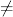```axiom)set output tex on
```
```axiom)set output algebra on
inequation(a,b)
(1)  a ~= b```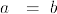(1)
Type: Inequation Symbol
```axiomt1:=inequation(2,3)
(2)  2 ~= 3```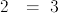(2)
Type: Inequation PositiveInteger?
```axiomt1::Boolean
(3)  true```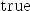(3)
Type: Boolean
```axiomt2:=equation(2,3)
(4)  2= 3```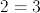(4)
Type: Equation PositiveInteger?
```axiomt2::Boolean
(5)  false```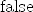(5)
Type: Boolean
```axioms1:= not t1
(6)  2= 3```(6)
Type: Equation PositiveInteger?
```axioms1::Boolean
(7)  false```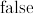(7)
Type: Boolean
```axiomt1*4
There are 34 exposed and 23 unexposed library operations named *
having 2 argument(s) but none was determined to be applicable.
Use HyperDoc Browse, or issue
)display op *
package-calling the operation or using coercions on the arguments
will allow you to apply the operation.
Cannot find a definition or applicable library operation named *
with argument type(s)
Inequation PositiveInteger
PositiveInteger
Perhaps you should use "@" to indicate the required return type,
or "\$" to specify which version of the function you need.
t1+t2
There are 15 exposed and 5 unexposed library operations named +
having 2 argument(s) but none was determined to be applicable.
Use HyperDoc Browse, or issue
)display op +
package-calling the operation or using coercions on the arguments
will allow you to apply the operation.
Cannot find a definition or applicable library operation named +
with argument type(s)
Inequation PositiveInteger
Equation PositiveInteger
Perhaps you should use "@" to indicate the required return type,
or "\$" to specify which version of the function you need.
t1*t2
There are 34 exposed and 23 unexposed library operations named *
having 2 argument(s) but none was determined to be applicable.
Use HyperDoc Browse, or issue
)display op *
package-calling the operation or using coercions on the arguments
will allow you to apply the operation.
Cannot find a definition or applicable library operation named *
with argument type(s)
Inequation PositiveInteger
Equation PositiveInteger
Perhaps you should use "@" to indicate the required return type,
or "\$" to specify which version of the function you need.```

```axiom)set output tex on
```
```axiom)set output algebra on
w1:=inequality(a,b)
(8)  a < b```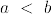(8)
Type: Inequality Symbol
```axiomw2:=inequality(2,3)
(9)  2 < 3```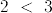(9)
Type: Inequality PositiveInteger?
```axiomw2::Boolean
(10)  true```(10)
Type: Boolean
```axiomnot w1
(11)  a >= b```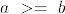(11)
Type: Inequality Symbol
```axiomw3:=not w2
(12)  2 >= 3```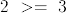(12)
Type: Inequality PositiveInteger?
```axiomw3::Boolean
(13)  false```(13)
Type: Boolean